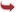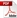International Journal of Scientific and Research Publications

#### IJSRP, Volume 9, Issue 8, August 2019 Edition [ISSN 2250-3153]Mathematical Estimation for Maximum Flow in Electricity Distribution Network by Ford-Fulkerson Iteration Algorithm
Myint Than Kyi, San San Maw, Lin Lin Naing
Abstract: In this paper, application of the graph theory to handle the function of power flow management will be introduced. Well known Ford-Fulkerson algorithm in graph theory is used to calculate the maximum flow in electricity distribution network. The maximum flow problem is one of the most fundamental problems in network flow theory and has been investigated extensively. The Ford-Fulkerson algorithm is a simple algorithm to solve the maximum flow problem and based on the idea of searching augmenting path from a started source node to a target sink node. It is one of the most widely used algorithms in optimization of flow networks and various computer applications. The implementations for the detail steps of algorithm will be illustrated by considering the maximum flow of proposed electricity distribution network in Kyaukse District, Mandalay Division, Myanmar as a case study. The goal of this paper is to find the maximum possible flow from the source node s to the target node t through a given proposed network.
[VIEW FULL PAPER][DOWNLOAD]

Reference this Research Paper (copy & paste below code):

Myint Than Kyi, San San Maw, Lin Lin Naing (2019); Mathematical Estimation for Maximum Flow in Electricity Distribution Network by Ford-Fulkerson Iteration Algorithm; International Journal of Scientific and Research Publications (IJSRP) 9(8) (ISSN: 2250-3153), DOI: http://dx.doi.org/10.29322/IJSRP.9.08.2019.p9229